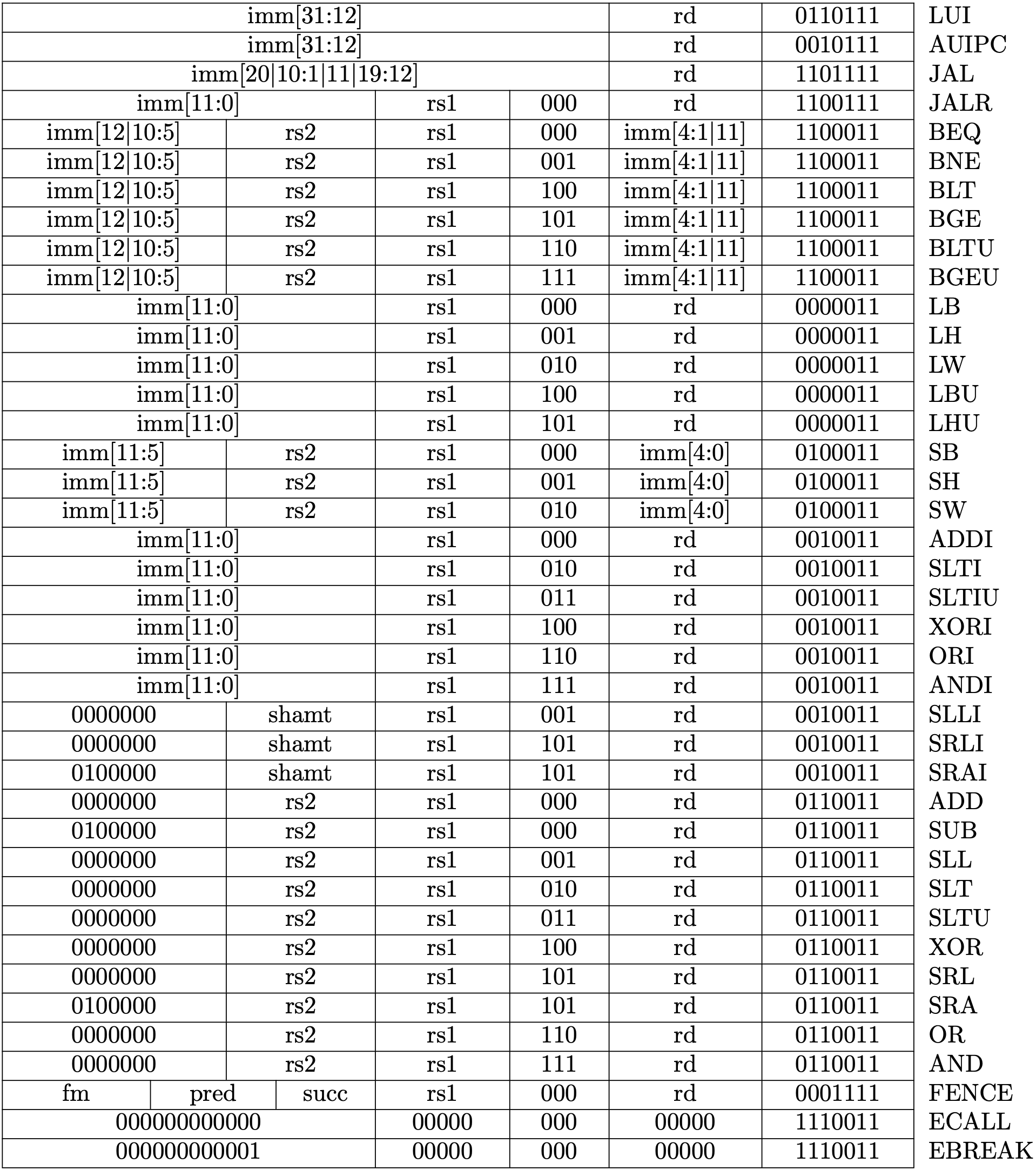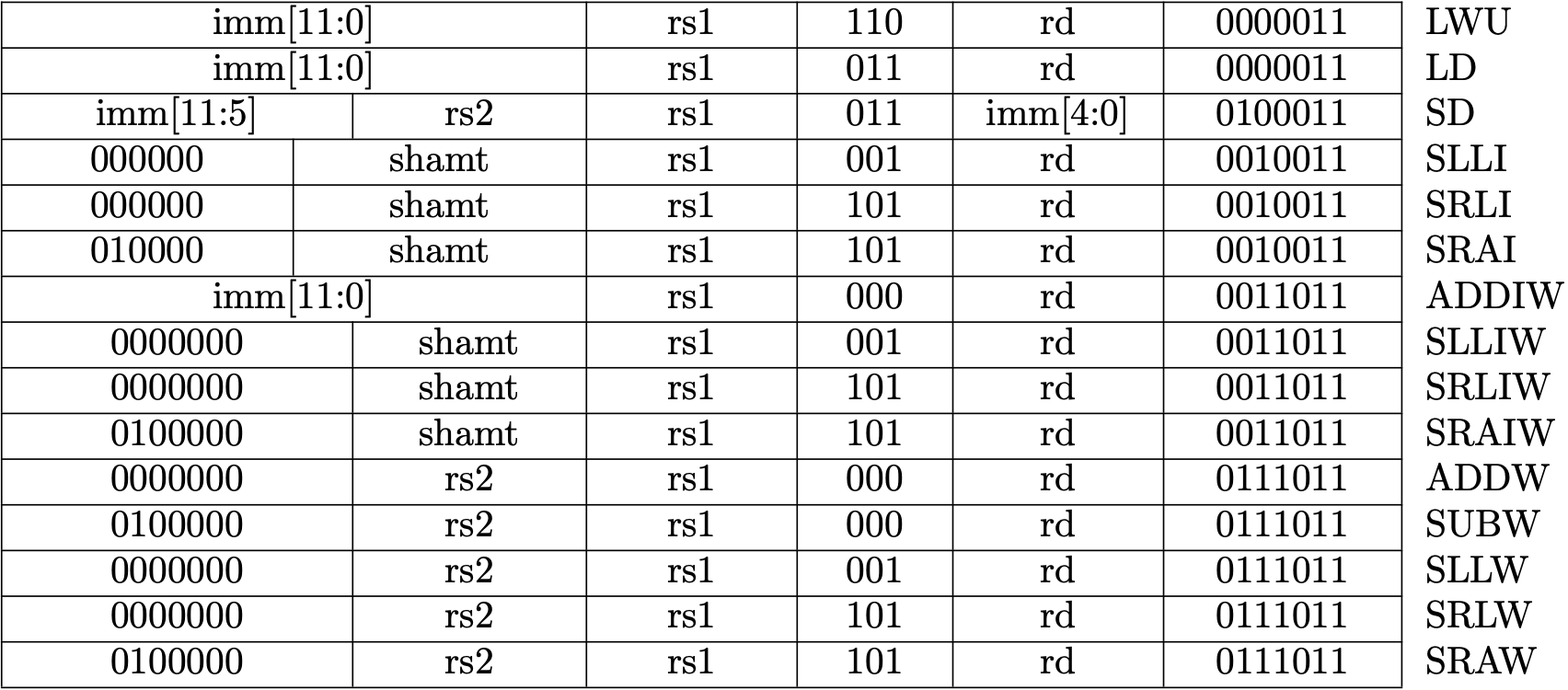# RV64I Base Integer Instruction Set

This is a part of Writing a RISC-V Emulator in Rust. Our goal is running xv6, a small Unix-like OS, in your emulator eventually.

This page will introduce the base integer instruction set for a 64-bit architecture (RV64I) all platforms must support. After implementing instructions in RV64I, We can execute the sample file that calculates a Fibonacci number in our emulator.

Sample binary files are available at d0iasm/rvemu-for-book/02/. We successfully see the result of the 10th Fibonacci number when we execute the sample binary file `fib.bin`.

``````// fib.c contains the following C code and fib.bin is the build result of it:
// int fib(int n);
// int main() {
//   return fib(10); // Calculate the 10th fibonacci number.
// }
// int fib(int n) {
//   if (n == 0 || n == 1)
//     return n;
//   else
//     return (fib(n-1) + fib(n-2));
// }

\$ cargo run fib.bin
...
x12=0x0 x13=0x0 x14=0x1 x15=0x37 // x15 should contain 55 (= 10th fibonacci number).
``````

## RV64I: Base Integer Instruction Set

RV64I is the base integer instruction set for the 64-bit architecture, which builds upon the RV32I variant. RV64I shares most of the instructions with RV32I but the width of registers is different and there are a few additional instructions only in RV64I.

The base integer instruction set has 47 instructions (35 instructions from RV32I and 12 instructions from RV64I). We've already implemented `add` and `addi` at 1.1 CPU with Two Instructions, load and store instructions at 1.2 Memory and System Bus, and `ecall` and `ebreak` at 1.4 Privileged Architecture. The remaining instructions are coverd by this page.

Fig 2.1 and Fig 2.2 are the lists for RV32I and RV64I, respectively. We're going to implement all instructions in the figures.Fig 1.1 RV32I Base Instruction Set (Source: RV32I Base Instruction Set table in Volume I: Unprivileged ISA)Fig 1.2 RV64I Base Instruction Set (Source: RV64I Base Instruction Set table in Volume I: Unprivileged ISA)

## Instructions List

The following table is a brief explanation for each instruction. The book won't describe the details of each instruction but will indicate points to be noted when you implement instructions. In addition, you can see the implementation in d0iasm/rvemu-for-book/02/src/cpu.rs and description in Chapter 2 RV32I Base Integer Instruction Set and Chapter 5 RV64I Base Integer Instruction Set in the unprivileged specification.

#### Points to be noted

• Arithmetic operations are done by wrapping functions to avoid an overflow.
• Sign-extended is done by casting from a smaller signed integer to a larger signed integer.
• The amount for 64-bit shift operations is encoded in the lower 6 bits in an immediate, and the amount for 32-bit shift operations is encoded in the lower 5 bits.
InstructionPseudocodeDescription
lui rd, immx[rd] = sext(imm[31:12] << 12)Load upper immediate value.
auipc rd, immx[rd] = pc + sext(imm[31:12] << 12)Add upper immediate value to PC.
jal rd, offsetx[rd] = pc + 4; pc += sext(offset)Jump and link.
jalr rd, offset(rs1)t = pc+4; pc = (x[rs1] + sext(offset)&~1); x[rd] = tJump and link register.
beq rs1, rs2, offsetif (rs1 == rs2) pc += sext(offset)Branch if equal.
bne rs1, rs2, offsetif (rs1 != rs2) pc += sext(offset)Branch if not equal.
blt rs1, rs2, offsetif (rs1 < rs2) pc += sext(offset)Branch if less than.
bge rs1, rs2, offsetif (rs1 >= rs2) pc += sext(offset)Branch if greater than or equal.
bltu rs1, rs2, offsetif (rs1 < rs2) pc += sext(offset)Branch if less than, unsigned.
bgeu rs1, rs2, offsetif (rs1 >= rs2) pc += sext(offset)Branch if greater than or equal, unsigned.
lb rd, offset(rs1)x[rd] = sext(M[x[rs1] + sext(offset)][7:0])Load byte (8 bits).
lh rd, offset(rs1)x[rd] = sext(M[x[rs1] + sext(offset)][15:0])Load halfword (16 bits).
lw rd, offset(rs1)x[rd] = sext(M[x[rs1] + sext(offset)][31:0])Load word (32 bits).
lbu rd, offset(rs1)x[rd] = M[x[rs1] + sext(offset)][7:0]Load byte, unsigned.
lhu rd, offset(rs1)x[rd] = M[x[rs1] + sext(offset)][15:0]Load halfword, unsigned.
sb rs2, offset(rs1)M[x[rs1] + sext(offset)] = x[rs2][7:0]Store byte.
sh rs2, offset(rs1)M[x[rs1] + sext(offset)] = x[rs2][15:0]Store halfword.
sw rs2, offset(rs1)M[x[rs1] + sext(offset)] = x[rs2][31:0]Store word.
slti rd, rs1, immx[rd] = x[rs1] < x[rs2]Set if less than.
sltiu rd, rs1, immx[rd] = x[rs1] < x[rs2]Set if less than, unsigned.
xori rd, rs1, immx[rd] = x[rs1] ^ sext(imm)Exclusive OR immediate.
ori rd, rs1, immx[rd] = x[rs1]sext(imm)
andi rd, rs1, immx[rd] = x[rs1] & sext(imm)AND immediate.
slli rd, rs1, shamtx[rd] = x[rs1] << shamtShift left logical immediate.
srli rd, rs1, shamtx[rd] = x[rs1] >> shamtShift right logical immediate.
srai rd, rs1, shamtx[rd] = x[rs1] >> shamtShift right arithmetic immediate.
sub rd, rs1, rs2x[rd] = x[rs1] - x[rs2]Subtract.
sll rs, rs1, rs2x[rd] = x[rs1] << x[rs2]Shift left logical.
slt rd, rs1, rs2x[rd] = x[rs1] < x[rs2]Set if less than.
sltu rd, rs1, rs2x[rd] = x[rs1] < x[rs2]Set if less than, unsigned.
xor rd, rs1, rs2x[rd] = x[rs1] ^ x[rs2]Exclusive OR.
srl rd, rs1, rs2x[rd] = x[rs1] >> x[rs2]Shift right logical.
sra rd, rs1, rs2x[rd] = x[rs1] >> x[rs2]Shift right arithmetic.
or rd, rs1, rs2x[rd] = x[rs1]x[rs2]
and rd, rs1, rs2x[rd] = x[rs1] & x[rs2]AND.
lwu rd, offset(rs1)x[rd] = M[x[rs1] + sext(offset)][31:0]Load word, unsigned.
ld rd, offset(rs1)x[rd] = M[x[rs1] + sext(offset)][63:0]Load doubleword (64 bits), unsigned.
sd rs2, offset(rs1)M[x[rs1] + sext(offset)] = x[rs2][63:0]Store doubleword.
slliw rd, rs1, shamtx[rd] = sext((x[rs1] << shamt)[31:0])Shift left logical word immediate.
srliw rd, rs1, shamtx[rd] = sext((x[rs1] >> shamt)[31:0])Shift right logical word immediate.
sraiw rd, rs1, shamtx[rd] = sext((x[rs1] >> shamt)[31:0])Shift right arithmetic word immediate.
subw rd, rs1, rs2x[rd] = sext((x[rs1] - x[rs2])[31:0])Subtract word.
sllw rd, rs1, rs2x[rd] = sext((x[rs1] << x[rs2][4:0])[31:0])Shift left logical word.
srlw rd, rs1, rs2x[rd] = sext(x[rs1][31:0] << x[rs2][4:0])Shift right logical word.
sraw rd, rs1, rs2x[rd] = sext(x[rs1][31:0] << x[rs2][4:0])Shift right arithmetic word.

### Fence Instruction

We won't explain `fence`. The `fence` instruction is a type of barrier instruction to apply an ordering constraint on memory operations issued before and after it. We don't need it since our emulator is a single core system and doesn't reorder memory operations (out-of-order execution).

## Testing

We're going to test instructions we implemented in this step by calculating a Fibonacci number and check if the registers are expected values. I prepared a sample binary file available at d0iasm/rvemu-for-book/02/. Download the fib.bin file and execute it in your emulator.

Calculating a Fibonacci number is actually not enough to test all RV64I instructions, so it perhaps be better to use riscv/riscv-tests to make sure if your implementation is correct. However, it's not obvious how to use riscv-tests so I'll skip to use the test in this book for the sake of simplicity. If you are interested in using riscv-tests, the test file in rvemu may be helpful.

``````// fib.c contains the following C code and fib.bin is the build result of it:
// int fib(int n);
// int main() {
//   return fib(10); // Calculate the 10th fibonacci number.
// }
// int fib(int n) {
//   if (n == 0 || n == 1)
//     return n;
//   else
//     return (fib(n-1) + fib(n-2));
// }

\$ cargo run fib.bin
...
x12=0x0 x13=0x0 x14=0x1 x15=0x37 // x15 should contain 55 (= 10th fibonacci number).
``````

### How to Build Test Binary

If you want to execute a bare-metal C program you write, you need to make an ELF binary without any headers because our emulator just starts to execute at the address `0x0` . The Makefile helps you build a test binary.

``````\$ riscv64-unknown-elf-gcc -S fib.c
\$ riscv64-unknown-elf-gcc -Wl,-Ttext=0x0 -nostdlib -o fib fib.s
\$ riscv64-unknown-elf-objcopy -O binary fib fib.bin
``````